# Isotopes

## Isotopes

• We have already studied Atomic Number and Atomic Mass number.Lets discuss about Important Term Isotopes.
• Isotopes is defined as Species having same atomic number but different atomic mass number
• Difference between the isotopes is due to the presence of different number of neutrons present in the nucleus
• Example - Isotopes of Hydrogen
99.985% of hydrogen atoms contain only one proton called protium . 0.015% contains two other isotopes deuterium & tritium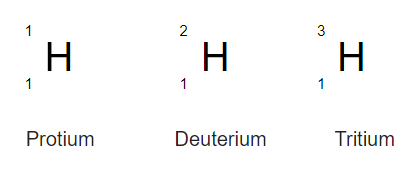• Chemical properties of atoms are controlled by the number of electrons. Number of neutrons present in the nucleus have very little effect on the chemical properties of an element.So, all the isotopes of a given element show same chemical behaviour.
• Isotopes of the elements have the naming convention of Element- except for hydrogen where specfic names is given to all the isotopes
Other Examples of Isotopes
a. Natural Carbon Isotopes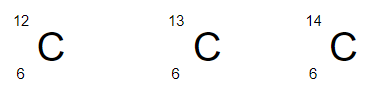12-C and 13-C are stable, occurring in a natural proportion of approximately 93:1. 14-C is produced by thermal neutrons from cosmic radiation in the upper atmosphere, and is transported down to earth to be absorbed by living biological material

b. Common Isotopes of Uranium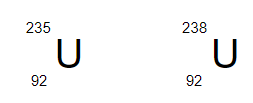The firs isotope 235-U is used un nuclear reactor and atomic bombs. Second isotope does not have much usage

c. Istotopes of Chlorine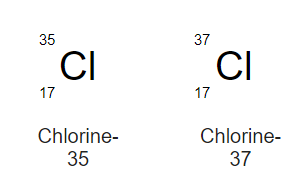Question 1
a. how many protons, neutrons, and electrons?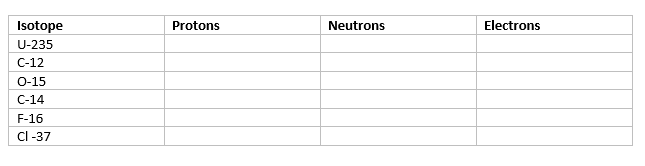b. Which one of the above is having the lowest and highest number of neutrons
Solution
a. We know that
Number of electrons= Number of Protons=Atomic Number
Number of neutrons= Atomic Mass Number - Atomic Number
Now we know that, Atomic Number of these isotopes are
Uranium = 92
Carbon =6
Oxygen= 8
Florine=9
Chlorine =17
Now Calculating based on the above formula, we can fill the table as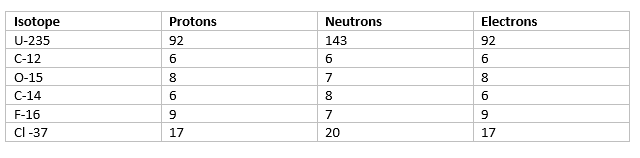b. From the table, it is clear that C-12 is having the lowest neutrons and U-235 is having the highest number of neutrons

Question 2
Identify the pairs which are not of isotopes?
(i) $^{12}_{6}X$,$^{13}_{6}Y$
(ii)$^{35}_{17}X$,$^{37}_{17}Y$
(iii) $^{14}_{6}X$,$^{14}_{7}Y$
(iv) $^{8}_{4}X$,$^{8}_{5}Y$
Solution
Isotopes have same Atomic Number,So (iii) and (iv) are not Isotopes

Question 3
In the below questions a statement of Assertion (A) followed by a statement of Reason (R) is given. Choose the correct option out of the choices
Assertion (A) : All isotopes of a given element show the same type of chemical behaviour.
Reason (R) : The chemical properties of an atom are controlled by the number of electrons in the atom.
(i) Both A and R are true and R is the correct explanation of A.
(ii) Both A and R are true but R is not the correct explanation of A.
(iii) A is true but R is false.
(iv) Both A and R are false
Solution
(i) is the correct option from definition

Question 4
Isotopes of an element have
(i)Similar chemical properties but different physical properties
(ii)Similar chemical and physical properties
(iii)Similar physical properties but different chemical properties
(iv) Different chemical and physical properties
Solution
(i) is the correct option

Question 5
Which property is different for neutral atoms of the two isotopes of the same element
(i)Number of protons
(ii)Atomic number
(iii)Number of neutrons
(iv)None of these
Solution
(iii) is the correct option

Question 6
Isotopes differ in
(i) Number of protons
(ii) Valency
(iii) Chemical reactivity
(iv) Number of neutrons
Solution
(iv) is the correct option